# Answers Solid State Test Paper – Class 12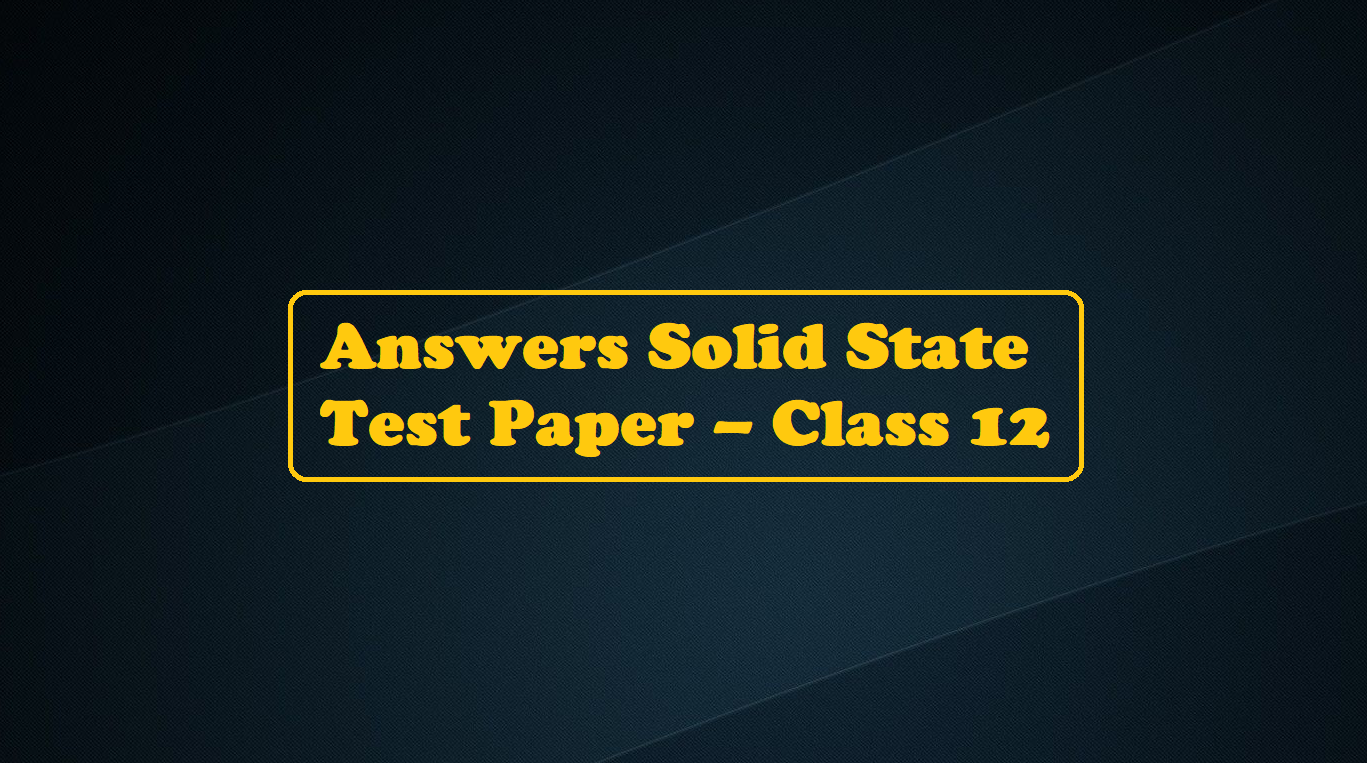# Answers Solid State Test Paper

Ans 1. (D).   Solid CO2 is an example of a molecular crystal.

Ans 2. (C).   Atoms along one edge or at corners do not touch each other in the fcc cell.

Ans 3. (D).

Ans 4.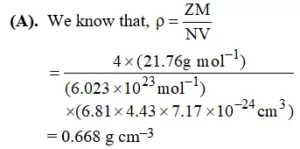Ans 5. (A).

Ans 6. (A).  n= N exp (-Ep/2kBT), where N = total atoms, n = Schottky defect are produced by removing n cations,           r = anions from the interior of the crystal.

Ans 7. (A).  The number of atoms per unit cell in simple, face-centered, and body-centered cubes is 1, 4, and 2.

Ans 8.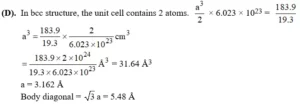Ans 9.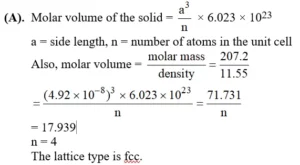Ans 10. (C).     Since tetrahedral holes are surrounded by 4 nearest neighbors. So, the C.N. of cation occupying tetrahedral hole is 4. Since the octahedral hole is surrounded by six nearest neighbors. So, the C.N. of cation occupying octahedral is 6. In Schottky, a pair of anion and cation leaves the lattice. So, the density of the lattice decreases.

Ans 11.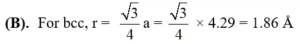Ans 12. (D).

Ans 13. (A). The spinel structure (Ab2O4) consists of an fcc array of O2- ion in which the A cation occupies one-eighth of the tetrahedral holes and the B cations the octahedral holes

Ans 14. (A). Equivalent points in unit cells of a periodic lattice lie on a Bravais lattice.

Ans 15.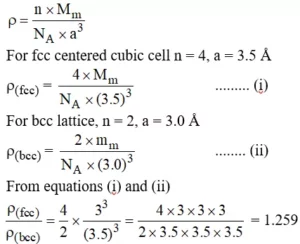Ans 16. (D). Anions occupy fcc positions and half of the tetrahedral holes are occupied by cations. Since there are four anions and 8 tetrahedral holes per unit cell, the fraction of volume occupied by spheres per unit volume of the unit cell is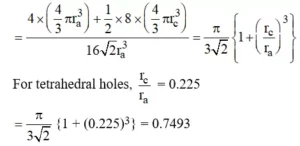Void volume =1 – 0.7493 = 0.2507/unit volume of unit cell.
% void space = 25.07%

Ans 17. (B).   Fused quartz is amorphous.

Ans 18. (B).   The structure of a compound of the formula A2B  in which each B2– ion is co-ordinated by eight A+ ions and each A+ ion by four B– ions is called an anti-fluorite structure.

Ans 19. (C).   The body diagonal respectively in a unit cell.
Their order is given by dfd > de > dfd .

Ans 20. (D). A crystal in which dipoles align themselves in an ordered way so as to give some net dipole moment, on applying a field the direction of polarization changes. Such crystals are called Piezoelectric crystals.

Ans 21. 1.

Ans 22. 8. Since the unit cell is fcc, the number of atoms should be 4, but since carbon atoms are arranged tetrahedrally, the atoms should also be present in alternate tetrahedral voids. The number of tetrahedral voids in fcc is 8 and hence the total number of carbon atoms per unit cell
(4 + 8) 1/2 = 8

Ans 23.Ans 24.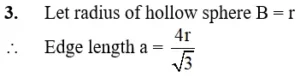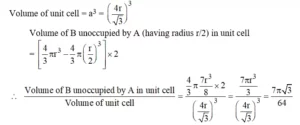Ans 25. 2.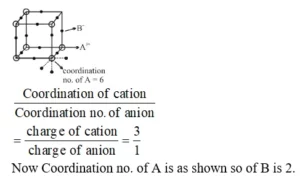Ans 26. T

Ans 27. T

Ans 28. F

Ans 29. T

Ans 30. F

Ans 31. T

Ans 32. F

Ans 33. T

Ans 34. F

Ans 35. T

Ans 36. T

Ans 37. T

Ans 38. T

Ans 39. T

Ans 40. F